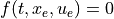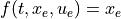# control.find_eqpt¶

control.find_eqpt(sys, x0, u0=None, y0=None, t=0, params=None, iu=None, iy=None, ix=None, idx=None, dx0=None, return_y=False, return_result=False)[source]

Find the equilibrium point for an input/output system.

Returns the value of an equilibrium point given the initial state and either input value or desired output value for the equilibrium point.

Parameters
• x0 (list of initial state values) – Initial guess for the value of the state near the equilibrium point.

• u0 (list of input values, optional) – If y0 is not specified, sets the equilibrium value of the input. If y0 is given, provides an initial guess for the value of the input. Can be omitted if the system does not have any inputs.

• y0 (list of output values, optional) – If specified, sets the desired values of the outputs at the equilibrium point.

• t (float, optional) – Evaluation time, for time-varying systems

• params (dict, optional) – Parameter values for the system. Passed to the evaluation functions for the system as default values, overriding internal defaults.

• iu (list of input indices, optional) – If specified, only the inputs with the given indices will be fixed at the specified values in solving for an equilibrium point. All other inputs will be varied. Input indices can be listed in any order.

• iy (list of output indices, optional) – If specified, only the outputs with the given indices will be fixed at the specified values in solving for an equilibrium point. All other outputs will be varied. Output indices can be listed in any order.

• ix (list of state indices, optional) – If specified, states with the given indices will be fixed at the specified values in solving for an equilibrium point. All other states will be varied. State indices can be listed in any order.

• dx0 (list of update values, optional) – If specified, the value of update map must match the listed value instead of the default value of 0.

• idx (list of state indices, optional) – If specified, state updates with the given indices will have their update maps fixed at the values given in dx0. All other update values will be ignored in solving for an equilibrium point. State indices can be listed in any order. By default, all updates will be fixed at dx0 in searching for an equilibrium point.

• return_y (bool, optional) – If True, return the value of output at the equilibrium point.

• return_result (bool, optional) – If True, return the result option from the `scipy.optimize.root()` function used to compute the equilibrium point.

Returns

• xeq (array of states) – Value of the states at the equilibrium point, or None if no equilibrium point was found and return_result was False.

• ueq (array of input values) – Value of the inputs at the equilibrium point, or None if no equilibrium point was found and return_result was False.

• yeq (array of output values, optional) – If return_y is True, returns the value of the outputs at the equilibrium point, or None if no equilibrium point was found and return_result was False.

• result (`scipy.optimize.OptimizeResult`, optional) – If return_result is True, returns the result from the `scipy.optimize.root()` function.

Notes

For continuous time systems, equilibrium points are defined as points for which the right hand side of the differential equation is zero:. For discrete time systems, equilibrium points are defined as points for which the right hand side of the difference equation returns the current state:.